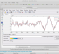## What are the wavelet packet functions and how are they different from the wavelet and scaling functions?Started by 3 weeks ago2 replieslatest reply 3 weeks ago79 views

I understand that in the decomposition process, wavelet and scaling functions are used to split the signal to approximation and detail coefficients.Most of the time I saw the wavelet and scaling function associated with the normal discrete wavelet transform. I assumed the same functions are used for the discrete wavelet packets but now both the approximation and details are decomposed to form a binary tree. The wavelet and scaling functions are set and have the same length regardless of the level of decomposition (based on the vanishing moment). I was looking into wavelet packets since I want coarser decomposition and came across the wavelet packet functionsand now I am confused as these functions remind me of the continuous wavelet transform and the scaling process of the wavelet functions. They appear to be more scaled relative to the depth but I might be wrong and do not have the same length (based on the vanishing moments).

So what are these wavelet packet functions and how are they used for the decomposition process?

[ - ]Hi,

You know in Continuous wavelet Transform, you scale wavelets. In decimated discrete wavelet transforms, after each filtering you downsample the signal by 2 factor afterwards. For the next level, when you apply same filter to this decimated signal, it is as if the filter is scaled by '2' relative to the signal.

What you have shown are reconstructed signals for each leaf of tree.

[ - ]